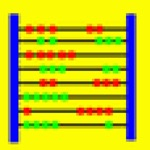# Abakus-Equa

Developed by K.-H. Erhard
USD 0.99

## App Details

Developer
K.-H. Erhard
Price
USD 0.99
Version
1.0
File Size
413.79 kB
Release Date
2011-03-19 22:48:22
Version Updated Date
2011-03-19 22:48:22
Languages
• English
compatibility
Minimum iOS Version 3.0 - iPhone - iPod - iPod - iPhone - iPhone - iPadWifi - iPad3G - iPodTouchThirdGen - iPhone4 - iPodTouchFourthGen - iPad2Wifi - iPad23G - iPhone4S - iPadThirdGen - iPadThirdGen4G - iPhone5 - iPodTouchFifthGen - iPadFourthGen - iPadFourthGen4G - iPadMini - iPadMini4G - iPhone5c - iPhone5s - iPhone6 - iPhone6Plus - iPodTouchSixthGen

## App Description

Abakus-Equa is a computer algebra program which performs
symbolic and numerical calculations on mathematical equations.

The current iOS version 1.0 contains the following functions:
- Exact solution of linear and non-linear equations
- Numerical solution of linear and non-linear equations
- Exact solution of systems of linear equations

For example, the input "ln(x+4) = 0" with variable "x" yields the output "x = -3".
The equation "7*cosh(x) = sinh(x)+9" resolves to "x = ln((9-sqrt(33))/6); x = ln((9+sqrt(33))/6)".
For the system of equations "x+2*y = 3; 4*x+5*y = 7",
Abakus-Equa calculates the exact solution "x = -1/3; y = 5/3".
The variable system "x+a*y = 2; x-y = 0" resolves to "x = y = 2/(1+a)".

Moreover Abakus-Equa contains a special editor for mathematical formulas.
So you can enter even complex expressions very fast.

Education

Education

Education